© | Dror Bar-Natan: Classes: 2009-10: AKT:

# Video AKT-091022-1

 width: 320 720 download ogg/AKT-091022-1_320.ogg For now, this video can only be viewed with web browsers that support

Videography by Karene Chu

Notes on AKT-091022-1:    [edit, refresh]

The Stonehenge Story to IHX and STU.

# Week of... Videos, Notes, and Links
: 3-colourings, Reidemeister's theorem, invariance, the Kauffman bracket.
: R23 invariance of the bracket, R1, the writhe, the Jones polynomial, programming the Jones polynomial.
Tricolourability
2 Sep 14 : More on Jones, some pathologies and more on Reidemeister, our overall agenda.
: The definition of finite type, weight systems, Jones is a finite type series.
: The skein relation for Jones; HOMFLY-PT and Conway; the weight system of Jones.
3 Sep 21 : FI, 4T, HOMFLY and FI and 4T, statement of the Fundamental Theorem, framed knots.
: Some dimensions of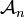${\mathcal A}_n$,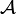${\mathcal A}$ is a commutative algebra,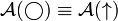${\mathcal A}(\bigcirc)\equiv{\mathcal A}(\uparrow)$.
Class Photo
:${\mathcal A}$ is a co-commutative algebra, the relation with products of invariants,${\mathcal A}$ is a bi-algebra.
4 Sep 28 Homework Assignment 1
Homework Assignment 1 Solutions
: The Milnor-Moore theorem, primitives, the map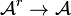${\mathcal A}^r\to{\mathcal A}$.
: Jacobi diagrams, AS, IHX, STU, and the equivalence of all that with 4T.
: The very basics on Lie algebras.
5 Oct 5 : Lie algebraic weight systems,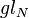$gl_N$.
: More on$gl_N$, Lie algebras and the four colour theorem.
: The "abstract tenssor" approach to weight systems,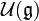${\mathcal U}({\mathfrak g})$ and PBW, the map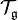${\mathcal T}_{\mathfrak g}$.
6 Oct 12 : Algebraic properties of${\mathcal U}({\mathfrak g})$ vs. algebraic properties of${\mathcal A}$.
Thursday's class canceled.
7 Oct 19 : Universal finite type invariants, filtered and graded spaces, expansions.
Homework Assignment 2
The Stonehenge Story
: The Stonehenge Story to IHX and STU.
: The Stonhenge Story: anomalies, framings, relation with physics.
8 Oct 26 : Knotted trivalent graphs and their chord diagrams.
: Zsuzsi Dancso on the Kontsevich Integral (1).
: Zsuzsi Dancso on the Kontsevich Integral (2).
9 Nov 2 : The details of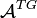${\mathcal A}^{TG}$.
: Three basic problems: genus, unknotting numbers, ribbon knots.
: The three basic problems and algebraic knot theory.
10 Nov 9 : Tangles and planar algebras, shielding and the generators of KTG.
Homework Assignment 3
No Thursday class.
11 Nov 16 Local Khovanov Homology
: Local Khovanov homology, I.
: Local Khovanov homology, II.
12 Nov 23 : Emulation of one structure inside another, deriving the pentagon.
: Peter Lee on braided monoidal categories, I.
: Peter Lee on braided monoidal categories, II.
13 Nov 30 : The relations in KTG.
: The Existence of the Exponential Function.
: The Final Exam, Dror's failures.
F Dec 7 The Final Exam on Thu Dec 10, 9-11, Bahen 6183.
Register of Good Deeds / To Do List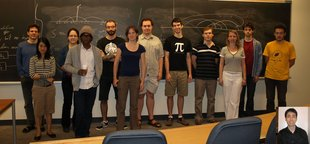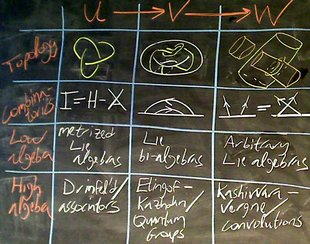refresh
panel
Managed by dbnvp: Click "Comment on h:mm:ss" below the video to add a comment on a specific time.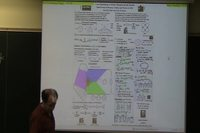0:05:10 [add] Handout glides to view 2: Astrology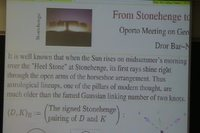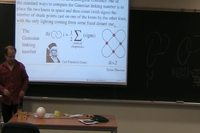0:11:03 [add] Handout glides to view 4: The Stonehenge Pairing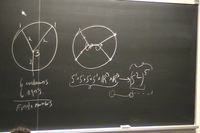0:25:28 [add] Handout glides to view 5: Z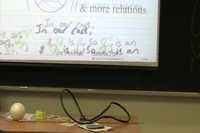0:32:47 [add] Handout glides to view 8: The Main Point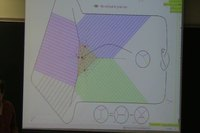0:45:48 [add] Handout glides to view 7: The Main Theorem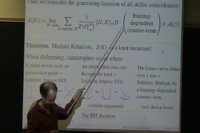0:46:08 [add] Handout glides to view 8: The Main Point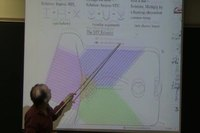0:46:12 [add] Handout glides to view 6: A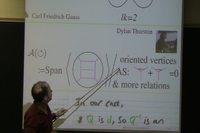0:46:15 [add] Handout glides to view 7: The Main Theorem